Reach Us+44-1474-556909
Generalizing of Differential Transform Method for Solving Nonlinear Differential Equations | OMICS International
Journal of Applied & Computational Mathematics
All submissions of the EM system will be redirected to Online Manuscript Submission System. Authors are requested to submit articles directly to Online Manuscript Submission System of respective journal.

# Generalizing of Differential Transform Method for Solving Nonlinear Differential Equations

Fabien Kenmogne*

Laboratory of Modelling and Simulation in Engineering and Biological Physics, Faculty of Science, University of Yaound´e I, Cameroon

*Corresponding Author:
Fabien Kenmogne
Laboratory of Modelling and Simulation in Engineering and
Biological Physics, Faculty of Science
University of Yaound’e I, Cameroon
Tel: (+237) 675 22 53 26
E-mail: [email protected]

Received October 17, 2014; Accepted November 24, 2014; Published December 16, 2014

Citation: Kenmogne F (2015) Generalizing of Differential Transform Method for Solving Nonlinear Differential Equations. J Appl Computat Math 4:196. doi: 10.4172/2168-9679.1000196

Copyright: © 2015 Kenmogne F. This is an open-access article distributed under the terms of the Creative Commons Attribution License, which permits unrestricted use, distribution, and reproduction in any medium, provided the original author and source are credited.

Visit for more related articles at Journal of Applied & Computational Mathematics

#### Abstract

In this paper, the differential transform method (DTM) is proved to be an excellent tool to investigate analytical and numerical solutions of nonlinear ordinary differential equations. The concept of differential transformation is briefly presented and some well-known properties of this DTM are rewritten in a more generalized forms. Several classes of nonlinear differential equations are solved analytically and numerically, in order to show the efficiency of the DTM, and the fundamental algorithms are presented in Matlab language.

#### Keywords

Differential transform method; Nonlinear ordinary differential equation; Exact solution; Numerical investigation

#### Introduction

Ordinary or partial differential equations are commonly encountered in several branches of Sciences including Biology, Physics, Chemistry and Mathematics [1-3]. They are particularly used in physical branches to describe qualitatively the dynamical behaviors of physical systems including the population growth, the motion of particles in potential fields, the signal voltage in electrical circuits, the dynamical behaviors of trees under the effects of wind [4- 7]. These differential equations obtained via physical laws (for e.g the Kirchhoff and Maxwell laws) are either linear or nonlinear. Although linear differential equations are easily solvable, nonlinear differential equations are difficult to solve and several of them do not admit analytical solutions and their solutions need to be approximated.

Many classical methods have been proposed to find exact or approximated solutions of these nonlinear differential equations, including the Lyapunov approach, the direct integration, the multiscale expansion technics, the harmonic balance method [1,8-10], the fractional homotopy analysis transform method based on the innovative adjustment in Laplace transform algorithm [11-13] and the differential transform method (DTM) [14,15]. The DTM has been used in recent years for solving nonlinear oscillator dynamics problems and this semi-exact method, based on the kth order derivative of state variables around the initial time t0 or space x0, does not need linearization .

It is important to mention that in the previous works in which the DTM is used, the initial time or space are chosen to be the origin (t0=0 or x0=0) and the obtained solutions which is in the Taylor series expansion form are compared to the known analytical solutions of the models equations, which are interesting but remain nevertheless unsatisfactory to study the influence of initial conditions on dynamical behaviors of nonlinear differential equations.

The motivation of this paper is to give an answer at the above problem. For this aim, the rest of the paper is organized as follows: Section (2) describes the DTM in which some modifications have been carried out, in order to take into account the initial conditions. In section (3), some analytical and numerical examples are presented to illustrate the efficiency of the DTM and the obtained numerical results are compared to the well-known four order Runge Kutta numerical scheme. Finally, we give the conclusion in Section (4).

#### Basic Concept of Differential Transform Method

The differential transformation method is one of the semianalytical method commonly used for solving ordinary and partial differential equations in the forms of polynomials as approximations of the exact solutions. The basic definition and the fundamental theorems of the DTM and its applicability for different kinds of differential equations are given in [14,16]. However in these works, all expansions are around the origin x0=0. Let us first remember that the differential transformation of the kth order derivative of any one dimensional variable y is given as follows: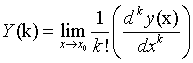(1)

If all values of the the transformation Y (k) are computed, one can find the expression of the variable y(x) by using the inverse transformation defined as follows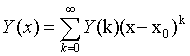(2)

It is obvious that the DTM satisfies the following set of conditions:

i) If y(x) = ay1(x) ± by2(x), then Y (k) = aY1(k) ± bY2(k), where a and b are any arbitrary constants.

ii)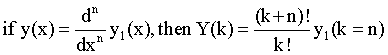iii)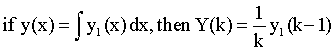iv)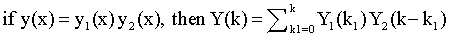v)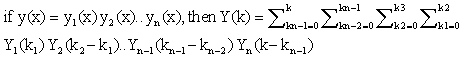All these five first theorems are well known and given in [14,16]. In addition to the above theorems, the last other theorems of the previous works are generalized for any given initial conditions as follows:

Vi)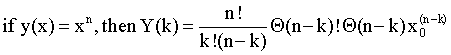where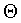is the step function,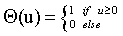(3)

and x0 the initial value of x. It is obvious that when x0=0, one recover the result obtained in [14,16] (that is Y (k)=δ(n−k)), where δ (u) is the Dirac delta function.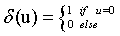(4)

Vii) If y(x)=exp(λx), then Y (k)=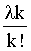exp(λx0), where λ is any arbitrary constant.

Viii) If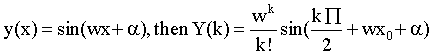where w and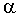are constants.

iX) if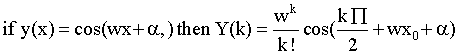X) If y(x) = a, then Y (k) = a δ (k), where a is a constant.

Xi)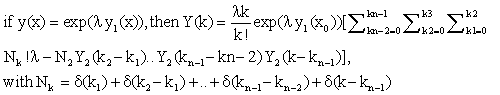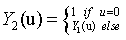(5)

The five firsts terms of Y (k) of this theorem are given in the extended form in the Appendix (5.1).

#### Applications of DTM to the Analytical and Numerical Results

In this section, some examples are given to illustrate the procedure outlined above.

The DTM in analytical results

The exact analytical solution of any set of solvable ordinary differential equations can be obtained by using properties i, ii, iii,..,xi and by choosing the initial differentiation point to be the origin x0=0. Let us first consider for illustrating the efficiency of the DTM, the following set of differential equations: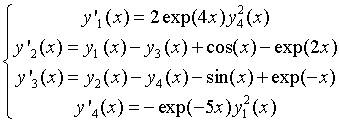(6)

and subjected to the initial conditions y1(0)=y2(0)=y4(0)=1, y3(0)=0, where the prime stands for the derivation with respect to variable x. Note that these equations under these initial conditions have exact analytical solutions in the form: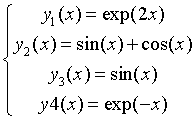(7)

By using the above properties around the expansion point x0=0, Equation(6) is transformed in differential form as follows: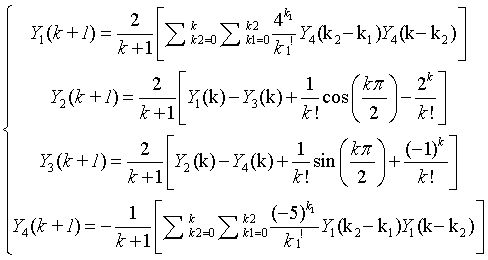(8)

and subjected to the initial condition: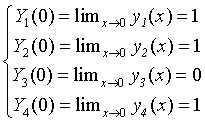(9)

From the above initial condition and accounting to the set of Equations. (8), the values of Yi(k), with i=1; 2; 3; 4 are progressively computed, leading to: Substituting the results of the above Table 1 into Equation(2) leads to the following solution of the set of Equations(6)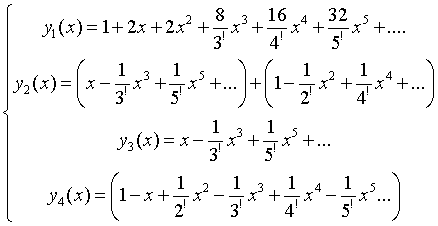(10)

Order of the differentiation Y1(k) Y2(k) Y3(k) Y4(k)
k=1 2 1 1 -1
k=2 2 -1/2 0 1/2
k=3 8/3 -1/3 -1/3 -1/3
k=4 16/4 -1/4 0 1/4
k=5 32/5 -1/5 -1/5 -1/5

Table 1: Table into Equation (2).

which coincide with solution (7) expanded as a power series near the origin x0=0.

The DTM in numerical investigations

As proved above, by considering the differential transformation near the constant and fixed initial value x0 (Figure 1), one obtains just the solution as a power series of the exact solution near this initial value. In order to seek the solution of the above problem with the DTM and valid for all values of x, we divide the space in small subspace, with space step h. To find the value of y(x+h) knowing the value of y(x), this method requires first the calculation of Y(k) at position x using the transformation (1) and the iterative process needs the value of y(x) at the initial space x=x0 and next, values of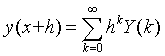(11)

Figure 1: Solution of Eq.(6). Solid line, exact solution of the system given by (7). Dash line, approximated solution (9) at the origin x=0. As one can see, they coincide only near the origin x=0.

As the differentiation is obtained here at kth order, with k → ∞, this method is accurate to (k + 1)th order in step size h, which tend to zero, leading this method more stable in numerical investigations as compared to other scheme. The proposed numerical technique that we can use to calculate Equation(11) is summarized in the flow chart shown in Figure 2. In what follows, the above example and two others are chosen to illustrate the procedure outlined in this subsection.

Example 1: Let us first consider the set of Equation(6), with the arbitrary initial conditions (y1(x0); y2(x0); y3(x0); y3(x0)), leading to the fact that the solution of this equation is not necessary given by (10). By using properties i, ii, iii,..,xi with now x0=0, instead of Equation(8), Equation(6) is now transformed in the following differential form: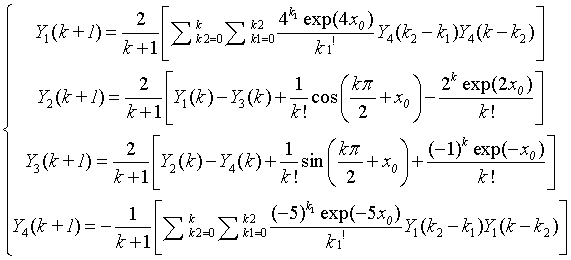(12)

which is well function of initial condition x0. This equation is subjected to the initial condition: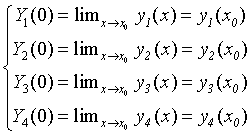(13)

By using the procedure (12) and the inverse DTM (11), the values of yi(x) can be progressively computed to give the profile of yi(x), which coincide with the solid line of Figure 3, which is the exact solutions given by Equation(7) if the initial conditions (9) are considered. In Matlab the algorithm may be given by the function DTMequation1 as given in Appendix(5.2):

Figure 3: Solution of Eq.(14) (a): Evolution of y(t), (b): phase space plot forthe following choice of parameters: a= 0:4, "=0:1, F=0:8. and w=1.

Example 2: The forced Vander Pol Duffing oscillator: Our second example is the forced Vander Pol Duffing oscillator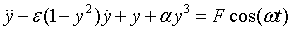(14)

where the dot stands for the derivation with respect to time t. This equation can be rewritten in the set of first order differential equations as follows: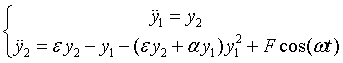(15)

with y1 ≡ y and y2=y?1,, and subjected to the initial condition y1(t0) and y2(t0). Using again the DTM, it is easy to show that the set of Equation(15) can be rewritten in the differential transformation form as: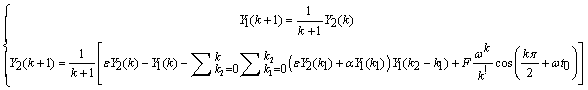(16)

As in the previous example, the values of y1(t) and y2(t) are progressively computed using both the procedure (16) and the inverse DTM (11), and the obtained results are plotted in Figure 4, which coincide exactly with results obtained by using the well-known four order Runge Kutta scheme. The Matlab algorithm used to compute yi(t) is now given by the function DTMequation2 as shown in Appendix(5.3):

Figure 4: Phase space plot of the solution of Eq.(14), obtained for the choiceof parameters: σ1=1:49, σ2 =0:872,=51:6, σ=255=256.

Example 3: Our third example is the following set of ordinary differential equation: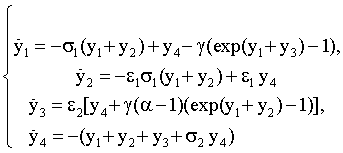(17)

subjected again to the initial condition y1(t0), y2(t0), y3(t0) and y4(t0), which can model several systems oscillators in which nonlinear diodes or nonlinear bipolar junction transistors are present, like the Colpitts oscillators [17,18]. This equation can be rewritten in the differential form as: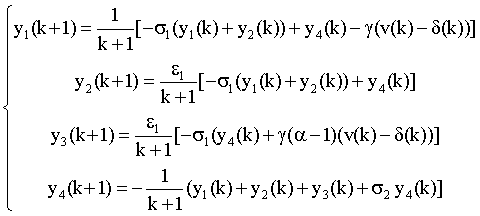(18)

where δ is again the delta function and where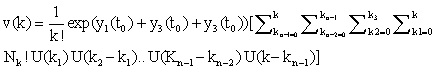(19)

With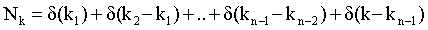and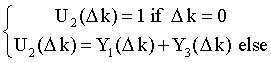(20)

In this work we have demonstrated the applicability of the differential transform method for solving analytically and numerically the set of nonlinear ordinary differential equations, with the help of some concretes examples. Firstly, we have ameliorated the existing properties of the DTM found in works preceding this work and we have next shown that the DTM is very powerful and efficient tool in finding exact solutions for a wide class of problems. The results of numerical simulation applied to certain classes of nonlinear differential equations show that the DTM is more accurate and the obtained solutions are very rapidly convergent in comparison with the traditional numerical schemes.

#### References

Select your language of interest to view the total content in your interested language

### Article Usage

• Total views: 15550
• [From(publication date):
February-2015 - Sep 17, 2019]
• Breakdown by view type
• HTML page views : 11195

## Post your commentCan't read the image? click here to refresh
###### Peer Reviewed Journals

Make the best use of Scientific Research and information from our 700 + peer reviewed, Open Access Journals

International Conferences 2019-20

Meet Inspiring Speakers and Experts at our 3000+ Global Annual Meetings

Top